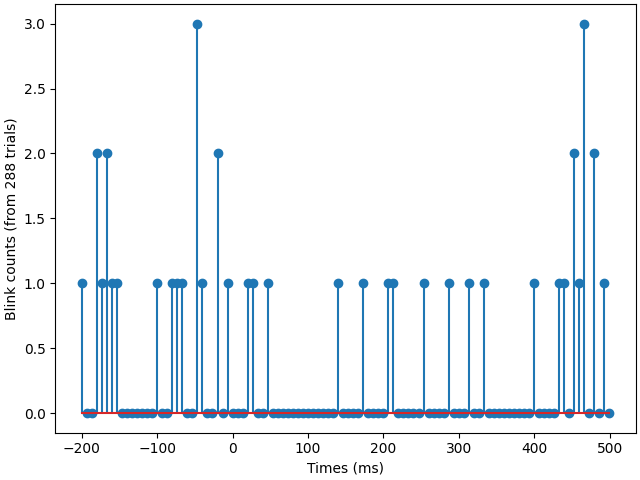# Show EOG artifact timing#

Compute the distribution of timing for EOG artifacts.

```# Authors: Eric Larson <larson.eric.d@gmail.com>
#
```
```import numpy as np
import matplotlib.pyplot as plt

import mne
from mne import io
from mne.datasets import sample

print(__doc__)

data_path = sample.data_path()
```

Set parameters

```meg_path = data_path / 'MEG' / 'sample'
raw_fname = meg_path / 'sample_audvis_filt-0-40_raw.fif'

# Setup for reading the raw data
events = mne.find_events(raw, 'STI 014')
eog_event_id = 512
eog_events = mne.preprocessing.find_eog_events(raw, eog_event_id)

picks = mne.pick_types(raw.info, meg=False, eeg=False, stim=True, eog=False)
tmin, tmax = -0.2, 0.5
event_ids = {'AudL': 1, 'AudR': 2, 'VisL': 3, 'VisR': 4}
epochs = mne.Epochs(raw, events, event_ids, tmin, tmax, picks=picks)

# Get the stim channel data
pick_ch = mne.pick_channels(epochs.ch_names, ['STI 014'])
data = epochs.get_data()[:, pick_ch, :]
data = np.sum((data.astype(int) & eog_event_id) == eog_event_id, axis=0)
```
```Opening raw data file /home/circleci/mne_data/MNE-sample-data/MEG/sample/sample_audvis_filt-0-40_raw.fif...
Read a total of 4 projection items:
PCA-v1 (1 x 102)  idle
PCA-v2 (1 x 102)  idle
PCA-v3 (1 x 102)  idle
Average EEG reference (1 x 60)  idle
Range : 6450 ... 48149 =     42.956 ...   320.665 secs
Reading 0 ... 41699  =      0.000 ...   277.709 secs...
319 events found
Event IDs: [ 1  2  3  4  5 32]
Using EOG channel: EOG 061
EOG channel index for this subject is: 
Filtering the data to remove DC offset to help distinguish blinks from saccades
Setting up band-pass filter from 1 - 10 Hz

FIR filter parameters
---------------------
Designing a two-pass forward and reverse, zero-phase, non-causal bandpass filter:
- Windowed frequency-domain design (firwin2) method
- Hann window
- Lower passband edge: 1.00
- Lower transition bandwidth: 0.50 Hz (-12 dB cutoff frequency: 0.75 Hz)
- Upper passband edge: 10.00 Hz
- Upper transition bandwidth: 0.50 Hz (-12 dB cutoff frequency: 10.25 Hz)
- Filter length: 1502 samples (10.003 sec)

[Parallel(n_jobs=1)]: Using backend SequentialBackend with 1 concurrent workers.
[Parallel(n_jobs=1)]: Done   1 out of   1 | elapsed:    0.0s remaining:    0.0s
[Parallel(n_jobs=1)]: Done   1 out of   1 | elapsed:    0.0s finished
Now detecting blinks and generating corresponding events
Found 46 significant peaks
Number of EOG events detected: 46
288 matching events found
Setting baseline interval to [-0.19979521315838786, 0.0] sec
Applying baseline correction (mode: mean)
4 projection items activated
Using data from preloaded Raw for 288 events and 106 original time points ...
```

Plot EOG artifact distribution

```fig, ax = plt.subplots()
ax.stem(1e3 * epochs.times, data)
ax.set(xlabel='Times (ms)',
ylabel='Blink counts (from %s trials)' % len(epochs))
fig.tight_layout()
```Total running time of the script: ( 0 minutes 3.387 seconds)

Estimated memory usage: 128 MB

Gallery generated by Sphinx-Gallery